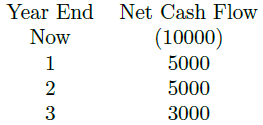# Tag: MCQs FM

## Mathematics of Finance – 3The Quiz about Mathematics of Finance for the preparation of Exams related to CA, CIMA, ICMAP, and MBA. MCQs covers many Business related fields (such as Business Administration, Commerce, and Charted Accountancy related Institutes) in which the subject of Business Mathematics is taught.

The quiz is about Mathematics of Finance which covers the topics of simple interest, compound interest, annuities, future values of annuity, the present value of an annuity, discounted cash flows, cash inflow, cash out flow, present values, and net present value.

1. A sum of Rs 10000 is invested in a saving account that pays interest at 10\% per annum compounded annually. If the amount is kept on deposit for 5 years, what will the compound amount equal to?

2. If the NPV is $\$928$when the discount rate is 10 percent and$-\$628$ when it is 20 percent, calculate the internal rate of return to two dp.

3. A bond increases from $\$3500$to$\$4000$ over 3 years. Complete the following calculation of the effective annual rate of interest: (i) Three-year ratio=?, (ii) Annual ratio=?, and (iii) Effect annul rate=? percent (on dp)

4. A building society adds interest monthly to accounts even though interest rates are expressed in annual terms. The current rate is stated as a 4.8 percent annum. If an investor deposits $\$2500\$ on 1 January, calculate the value of the account on 31 August, giving your answer correct to the nearest Penny.

5. If interest is compounded monthly, what total sum will have accumulated after five complete years?

7. A man received RS 100000 from his friend at 10% per year on simple interest. How much will he pay after 5 years?

8. A company is planning capital investment for which the following year-end cash flows have been estimatedUse tables to calculate the net present value (NPV) of the project using tables if the company has a cost of capital of 15 percent.

9. A man wants to sell his laptop. There are two offers, one at Rs 10000 cash and the other on the credit of Rs 11800 to be paid after one year. Money could be invested at 18% per annum compounded annually. Which is the better option?

10. What is the simple interest rate of a principle amount of RS 1500 which grows to Rs 1725 after five years?

11. The amount of Rs 7500 at compound interest rate of 4\% per annum for 2 years would be _________

12. Rs. 5000 will give Rs 500 as simple interest at the rate of 5% per annum after __________ years.

13. At what annual rate of compound interest will Rs 20000 grow to Rs 27210 after four years?

14. The net present value (NPV) of a project is RS 25000 when the discount rate is 15%. Which one of the following statements is correct on the basis of the information given?

15. Ali borrowed Rs 5000 from Akhter at simple interest. After 3 years, Akhter got Rs 300 more than what he had given to Ali. What was the rate of interest per annum?

16. A sum of money placed at compound interest doubles itself in 5 years. It will amount to eight times in:

17. The net present value at a discount rate of 12% is Rs -2000 and at 11% it is Rs 2808. Which one of the following statements about the internal rate of return (IRR) is correct?

18. What amount of money should be invested for 5 years to get a sum of Rs 1000000. If interest is compounded half-yearly at the rate of 8% per annum?

19. A man borrowed Rs 800 at 10% per annum simple interest and immediately lent the whole sum at the rate of 10% per annum compounded annually. What does he gain at the end of the 2nd year?

20. Mrs. Shahida invested her entire savings of Rs 15000 at a simple interest rate of 10%. How many years she would have to wait in order to double his amount?